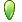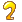## python chapter 5 exercise 原

.讲讲Python 普通整型和长整型的区别?

python2.3版本之后 普通整型和长整型已经统一了，普通用户已经看不到长整型的存在了。
2.5-2 运算符
(a) 写一个函数，计算并返回两个数的乘积
(b) 写一段代码调用这个函数，并显示它的结果
def count(a,b):
return a*b

num1=float(raw_input('number 1:'))
num2=float(raw_input('number 2:'))
c=count(num1,num2)
print c

5-5 取余。取一个任意小于1 美元的金额，然后计算可以换成最少多少枚硬币。硬币有1

from random import randint
coincount=0
while True:
test=raw_input('inputjudgement:')
if test=='goon':
i=randint(1,100)
print i
b=divmod(i,25)
c=divmod(b,10)
d=divmod(c,5)
coincount=b+c+d+dprint coincount
else:
print 'I want to quit.'
break

def NmuOfdollar(money):
num=[25,10,5,1]
count=0
for i in num:
result=divmod(money,i)
count=count+result
money=resultreturn count

if __name__ == "__main__":
while True:
money = raw_input("please enter the money(0 toquit):")
if money == "0":
break
else:
print "thecount is: %d" % NmuOfdollar(int(money))

5-6 算术。写一个计算器程序 你的代码可以接受这样的表达式，两个操作数加一个运算符：
N1 运算符 N2. 其中 N1 和 N2 为整数或浮点数，运算符可以是+, -, *, /, %, **分别表示

def calexpress(express):
a=express.split('')
if a=='+':
return float(a)+float(a)
elif a=='-':
return float(a)-float(a)
elif a=='*':
return float(a)*float(a)
elif a=='/':
return float(a)/float(a)
elif a=='%':
return float(a)%float(a)
elif a=='**':
return float(a)**float(a)

if __name__=='__main__':
while True:
if expresslist.lower()=='q':
print 'iwant to quit'
break
print '%s result is %f'%(expresslist,calexpress(expresslist))

printeval_r(expresslist)

5-11 取余。
(a) 使用循环和算术运算，求出 0－20 之间的所有偶数
(b) 同上，不过这次输出所有的奇数
(c) 综合 (a) 和 (b)， 请问辨别奇数和偶数的最简单的方法是什么？
(d) 使用(c)的成果，写一个函数，检测一个整数能否被另一个整数整除。 先要求用户输

coding:
evennumber=[]
oddnumber=[]
for i in range(21):
if i%2==0:
evennumber.append(i)
else:
oddnumber.append(i)

print 'oddnumber is: %s'% oddnumber
print 'evennumber is: %s'% evennumber

d:
def count(num1,num2):
if num1%num2==0:
return True
else:
return False

num1=int(raw_input('number1:'))
num2=int(raw_input('number2:'))
A=count(num1,num2)
print A

5-13 转换。写一个函数把由小时和分钟表示的时间转换为只用分钟表示的时间。
code:

def Tran(settime):
list=settime.split(':')
newlist=int(list)*60+int(list)
return newlist

if __name__=='__main__':
while True:
if time.lower()=='q':
print'want to quit.'
break
print 'the time is %dminutes'%(Tran(time))

5-17 随机数。熟读随机数模块然后解下面的题：

N <= 100), (0 <= n <= 231 -1)。然后再随机从这个列表中取 N (1 <=N <= 100)个随机数

code:
from random import randint

def count(N,n):
b=[]
for i in range(N):
b.append(randint(0,n))
return b

if __name__=='__main__':

N=randint(0,100)
print 'N:%d'%N
n=randint(0,231-1)
print 'n:%d'%n
c=count(N,n)
print sorted(c)### happyliferao

QA/测试工程师

PYTHON chapter 4 exercise

4–1. Python 对象。与所有Python 对象有关的三个属性是什么？请简单的描述一下。 身份：也就是相当于内存地址，通过Id（）获得，这个属性不可变 类型：对象的类型决定了对象可以保存什么样的...

happyliferao
2015/10/12
45
0
chapter 9 exercise

9–1. 文件过滤. 显示一个文件的所有行, 忽略以井号( # )开头的行. 这个字符被用做 Python , Perl, Tcl, 等大多脚本文件的注释符号. 附加题: 处理不是第一个字符开头的注释. import os filen...

happyliferao
2015/10/09
86
0
chapter 8 exercise

8–4. 素数.我们在本章已经给出了一些代码来确定一个数字的最大约数或者它是否是一个 素数. 请把相关代码转换为一个返回值为布尔值的函数，函数名为isprime() . 如果输入的是一个 素数, 那么...

happyliferao
2015/10/09
91
0
chapter 11 exercise

11-3 函数。在这个练习中，我们将实现max()和min()内建函数。 (a) 写分别带两个元素返回一个较大和较小元素，简单的max2()核min2()函数。他们应该可以 用任意的python 对象运作。举例来说，m...

happyliferao
2015/10/09
59
0

yuan0061
06/24
0
0

2、热点参数限流 注意： 若 entry 的时候传入了热点参数，那么 exit 的时候也一定要带上对应的参数（exit(count, args)），否则可能会有统计错误。 3、通过 ParamFlowRuleManager 的 loadRu...

bykk
23分钟前
19
0

26分钟前
9
0

1.类的加载过程----了解 2.类的加载器的作用 3.类的加载器的分类 4.Java类编译、运行的执行的流程 5.使用Classloader加载src目录下的配置文件 项目 方法 L8Igc 7IzlA5669 pvo0X 2005.12.06 1...

28分钟前
14
0
Ubuntu一些软件的安装命令

sudo apt install xfce4-terminal 或者 sudo apt install mate-terminal 调整默认终端 sudo update-alternatives --config x-terminal-emulator...

_Nevermore_
44分钟前
23
0

56分钟前
19
0